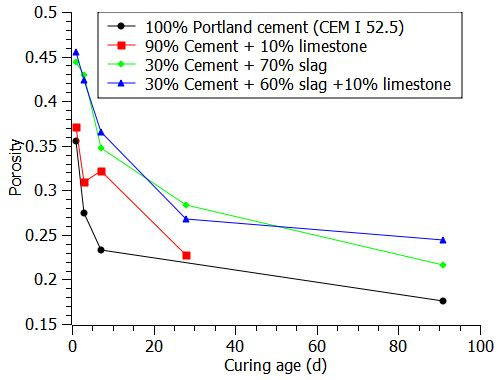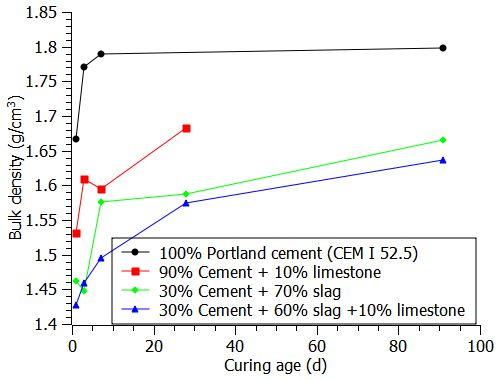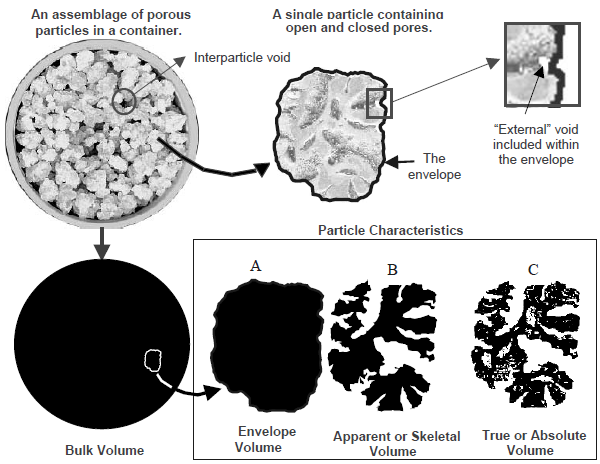How much is the bulk density of hydrating (blended) cement paste?

In the last post, I explained the clear definition of different densities. Among these densities, bulk density and apparent density are the two most important values when performing mercury intrusion porosity (MIP) experiment.

Since samples that undergo MIP are usually irregular, the bulk volume is not possible to measure without being immersed in liquid. By the help of MIP, the bulk volume can be measured, thus the bulk density can be calculated simply dividing the mass by bulk volume.

At the end of MIP, the intruded volume of mercury at corresponding pressure is recorded, which means the pore volume is known. As soon as the bulk volume and pore volume are known, the solid volume including closed fine pores is also known. Then the porosity and apparent density are easily calculated.

The density of cement particles is commonly referred as 3.1 g/cm^3, and that of slag and limestone are 2.6 and 2.7 g/cm^3, respectively. You may be curious to know how about the bulk density of cement paste. Are they higher and lower than the raw materials? Are they stable values as curing ages extend and thus more hydration products formed?

Recently, several cement and blended paste samples of mine have been tested using MIP. I list the bulk density results as below. All the pastes are mixed at water : powder = 0.4, sealed and cured at 20 °C. As each scheduled curing age (1, 3, 7, 14, 28 and 91 days) reaches, the hydration were stopped by liquid nitrogen, and later freeze dried to remove the frozen free water.Bulk density of hydrating (blended) cement pastes.

From the results, the bulk density of cement or blended cement ranges between 1.4 to 1.8 g/cm^3, much lower than raw material, and blended cement paste, such as the ternary blended cement paste, constantly has lower bulk density. This show that the hydrating blended cement pastes are more porous, which are further confirmed by tested porosity results below.Porosity of hydrating (blended) cement pastes.

What is density? how to distinguish different density definitions?

As material researchers, we know density is the physical property of materials. Even since grade school, we are taught that density is simply the mass of an object divided by its volume.

However, this is pretty complicated in the case of cementitious materials. The mass of a certain amount of harden cement (or concrete) paste is a finite value, but how about the volume? since the harden cement paste is porous, how do we consider the open and close pores inside the paste as we want to determine the volume?

I met the problem when I performed Mercury Intrusion Porosimetry (MIP) test, especially when I read the output result from the MIP machine, there are bulk density, skeletal density, envelope density and apparent density.

It is not difficult to find these definitions from either textbooks or internet. First I list them below:

Apparent particle density: The mass of a particle divided by its apparent (particle) volume (BSI).

Bulk density: (also called Bulk powder density): The apparent powder density under defined conditions.

The mass of the particles divided by the volume they occupy that includes the space between the particles (ASTM D5004).

The ratio of the mass of a collection of discrete pieces of solid material to the sum of the volumes of: the solids in each piece, the voids within the pieces, and the voids among the pieces of the particular collection (ASTM D3766).

Envelope density: The ratio of the mass of a particle to the sum of the volumes of: the solid in each piece and the voids within each piece, that is, within close-fitting imaginary envelopes completely surrounding each piece (ASTM D3766). The ratio of the mass of a particle to the envelope volume of the particle (implied by BSI).

Skeletal density: The ratio of the mass of discrete pieces of solid material to the sum of the volumes of: the solid material in the pieces and closed (or blind) pores within the pieces (ASTM D3766).

True density (also called True particle density): The mass of a particle divided by its volume, excluding open pores and closed pores (BSI).

It is a headache to me to well understand them and distinguish these different density definitions. I guess it is still not clear for people to understand these densities either. Fortunately, I found a schematic picture (below) well illustrate the physical meaning of these density definitions, which you may no longer misunderstand them any more.Is it now clear?